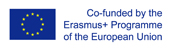This project has been funded with support from the European Commission.
This web site reflects the views only of the author, and the Commission cannot be held responsible for any use which may be made of the information contained therein.

Select language   >   IT EN RO PL LT

### Pythagoras theorem

Subject taught
- Math

Type of Product
- Web Site/Portal

Language Skills Developed
- Speaking
- Writing

Transferable/Scientific Skills Developed
Student will develop self-motivation, analytical skills, problem-solving skills.

Description
General aims:

Student will be able to explain visually and theoretically how Pythagoras theorem works, how to use it and how to proof it.
Student will be able to solve basic mathematical problems connected with Pythagoras theorem which can be used in the real life. He will know basic vocabulary in English connected with geometry. Student will develop positive learning habits such as organisational and problem-solving skills.

Linguistic aims:
- to read English with ease
- to understanding content
- to learn from written texts
- to master tools for gathering information
- to express understanding and experiences
- to enable students to understand topic correctly
- to translate words connected with percentages in English into the mother tongue.

Subject specific aims:
- student can explain visually and theoretically how Pythagoras theorem works
- student is able to use Pythagoras theorem in math problems which are connected directly with a right triangle
- student knows how to proof Pythagoras theorem
- student can solve problems using Pythagoras theorem even if they aren´t directly connected to a right triangle.

Target group age:
Students from primary school 13-14 years old. What is more, this web site can be used by anyone who wants to do extend one’s knowledge about Pythagoras theorem .

Level of competence in English (CEFR)
B1/B1+

Time required to use the resource with the students:
The material can be divided into three parts. This way the teacher can use the resource with the students for three lessons.

How to use it
The teacher can ask the students to familiarize themselves with the the first part of website at home. In this part the students can learn how how Pythagoras theorem works visually and theoretically and they will know a brief history about the theory.

Students should try to understand the text in English as well as the concept of the Pythagorean theorem and the content of the website.

During lessons the teacher can ask the student in English:
“What is the the Pythagorean theorem about?”,
“What is the history of this theorem?”,
“How to proof it?”
The students give the answers with the examples. The teacher should point the good answers on the web site and show students the examples from the resource to visualize the most important issues and definitions for example a Pythagorean triple. The teacher highlights some new words for students in English and students should find the translation these words into the mother tongue and write down the new words in English in the notebook with the right translation.
Then, the students do the basic examples where Pythagoras theorem is used. They are on the website in section two. Students will need to find the length of the hypotenuse or leg in a right triangle, check if a triangle is right, calculate the diagonal distance across a square.
They will also find out special types of right triangles, 30°-60° and 45°-45° right triangles. The teacher may need another lesson to finish the explanation of all examples from the section two. It would be also recommended to give students more examples connected with ones presented in section two.
The third lesson can be connected with the third section from the resource called “More advanced examples.” The students can discussed the problems in pairs and after that tried to express their conclusions and possible questions. The teacher comments and corrects their statements. Then, the student can solve similar math problems. After doing and checking the exercises teacher can ask students what they learn and ask them to write down in their notebooks: the distance formula and the formula for the diagonal distance of a cube.
The teacher can recommend and encourage the students to get to know more about Pythagorean theorem using other pages of the resource ( section four called “Continue to learn more about the Pythagorean theorem”).
Possible difficulties for the students
Students who have poor knowledge of English can have problems with understanding the content. Students who has problems doing basic operations with powers and roots can face difficulties in solving math problems with the Pythagorean theorem which are introduced on the web site which is recommended.